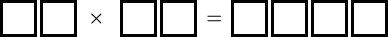#### You may also like### Lastly - Well

What are the last two digits of 2^(2^2003)?### Counting Factors

Is there an efficient way to work out how many factors a large number has?### Summing Consecutive Numbers

15 = 7 + 8 and 10 = 1 + 2 + 3 + 4. Can you say which numbers can be expressed as the sum of two or more consecutive integers?

# Times Right

##### Age 11 to 16 Challenge Level:Fill these boxes with the digits 1, 2, 3, 4, 5, 6, 7 and 8 using each digit only once, so that the expression is correct. How many different solutions can you find?

Is it possible to do this with the digits 0 to 7? What about using the digits 2 to 9?